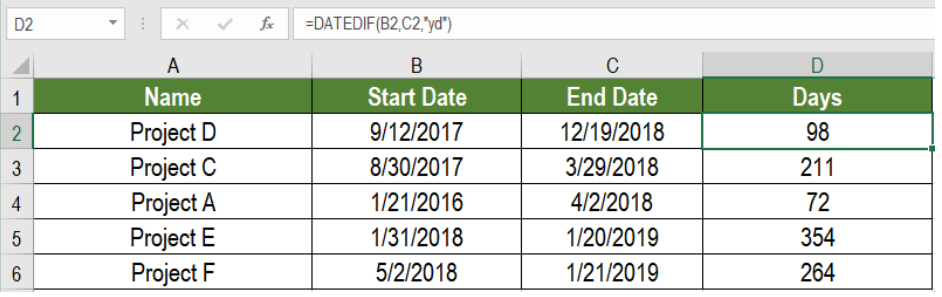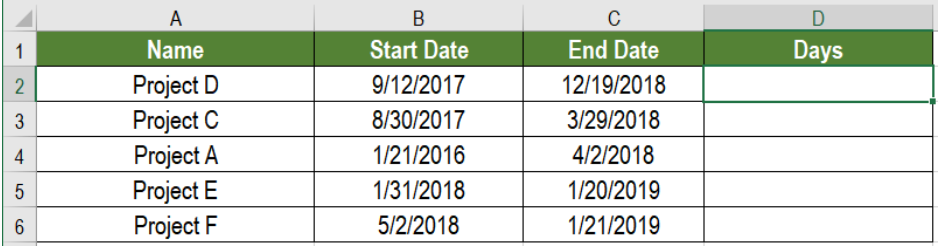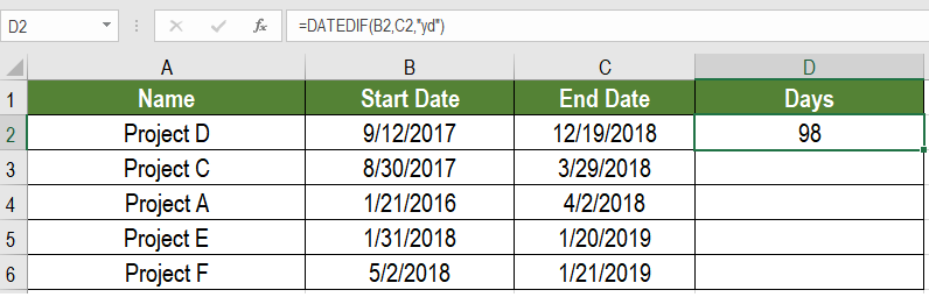Get instant live expert help with Excel or Google Sheets“My Excelchat expert helped me in less than 20 minutes, saving me what would have been 5 hours of work!”

#### Post your problem and you’ll get expert help in seconds.

Your message must be at least 40 characters
Our professional experts are available now. Your privacy is guaranteed.

# Get Days Between Dates Ignoring Years

Excel has made working with dates easier than ever before. We can perform some very handy tasks with dates. One such trick is to find days between two dates ignoring the year. DATEDIF helps us do this very easily. In this tutorial, we will learn how to get days between dates ignoring years.Figure 1. Example of How to Get Days Between Dates Ignoring Years

## General Formula

`=DATEDIF(start date,end date,"yd")`

## Process

This formula is based on the function DATEDIF. It calculates the days,months or years between two dates. It can represent this difference between two dates in a numbers of ways. DATEDIF uses three arguments. They are: start date, end date, and unit. We provide the start and end dates with the unit and Excel displays the difference.

The unit argument controls the representation of the result. To get days between dates ignoring years, we use the “yd” unit here. It returns the days ignoring years.

## Setting up Data

The following example uses a project information database. Column A, B and C has the project names, start date and end dates.Figure 2. The Sample Data Set

To find the days to complete the projects ignoring years in column D:

• We need to select cell D2 with our mouse.
• Assign the formula `=DATEDIF(A2,B2,”yd”) `to D2.
• Press Enter to apply the formula to D2.Figure 3. Applying the Formula to the Data

• Drag the formula from cells D2 to D6 to copy the formula to the entire column.

This will make column D display the days between the dates ignoring years.

Most of the time, the problem you will need to solve will be more complex than a simple application of a formula or function. If you want to save hours of research and frustration, try our live Excelchat service! Our Excel Experts are available 24/7 to answer any Excel question you may have. We guarantee a connection within 30 seconds and a customized solution within 20 minutes.

### Did this post not answer your question? Get a solution from connecting with the expert.Another blog reader asked this question today on Excelchat:
Solution examplesI need a datedif function that calculates the difference in 2 dates and if it returns more than 5 years, I need it to say yes and if not no.
Solved by O. Y. in 14 minsHi there. I need a column to automatically calculate the numbers of days from a start time to today's date.
Solved by T. F. in 16 minsi have this formula currently which works but not if I want it for every day - any ideas ?? (NETWORKDAYS.INTL(L1362,M1362,11,BH!A:A)-1)*("17:30"-"8:30")+IF(NETWORKDAYS.INTL(M1362,M1362,11,BH!A:A),MEDIAN(MOD(M1362,1),"8:30","17:30"),"17:30")-MEDIAN(NETWORKDAYS.INTL(L1362,L1362,11,BH!A:A)*MOD(L1362,1),"8:30","17:30")
Solved by A. D. in 28 mins## Subscribe to Excelchat.coAnother blog reader asked this question today on Excelchat: